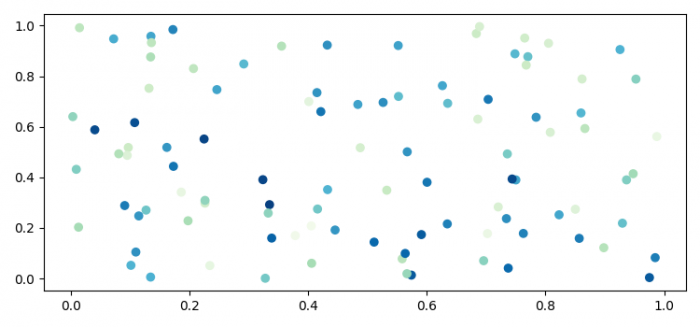# How to convert data values into color information for Matplotlib?

MatplotlibPythonData Visualization

To convert data values into color information for Matplotlib, we can take the following steps −

• Set the figure size and adjust the padding between and around the subplots.
• Get a colormap instance, defaulting to rc values if *name* is None.
• Create random values that could be converted into color information.
• Create random data points, x and y.
• Use scatter() method to plot x and y.
• To display the figure, use show() method.

## Example

import numpy as np
import matplotlib.pyplot as plt

plt.rcParams["figure.figsize"] = [7.50, 3.50]
plt.rcParams["figure.autolayout"] = True

plasma = plt.get_cmap('GnBu_r')
values = np.random.rand(100)

x = np.random.rand(len(values))
y = np.random.rand(len(values))

sc = plt.scatter(x, y, c=[plasma(val) for val in values])

plt.show()

## Output